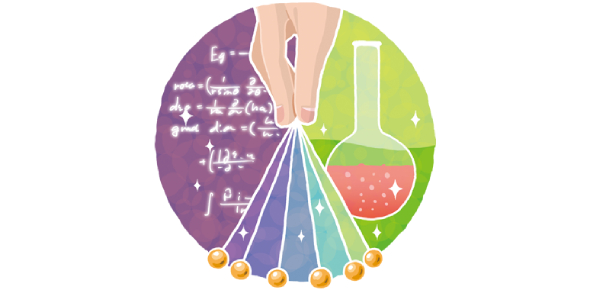# Basic Science Test: Quiz!

17 Questions | Total Attempts: 2069SettingsDo you like researching? Are you curious by nature, who always need scientific proof for every phenomenon? If you fall into this category, then congrats, you're a 'Science lover. ' This quiz will test your knowledge of biology, physics, computer science, chemistry, and astronomy. There is a list of 17 basic questions. Read the questions carefully and prove your worth.

• 1.
A helium nucleus being ejected from the nucleus of an atom can be referred to as:
• A.

Alpha Decay

• B.

Beta Decay

• C.

Gamma Decay

• D.

Fusion

• E.

• 2.
Which is the best example of convergent evolution.
• A.

Chimpanzees and Humans

• B.

Chimpanzees and Gorillas

• C.

Dolphins and Whales

• D.

Dolphins and Sharks

• E.

Dolphins and Humans

• 3.
Up, Down, Strange, Charmed
• A.

Baryons

• B.

Leptons

• C.

Positrons

• D.

Quarks

• E.

Tachyons

• 4.
The number of transistors that can be inexpensively placed on an integrated circuit is increasing exponentially, doubling approximately every two years.
• A.

Exponential Transistor Theory

• B.

Costanza's Law

• C.

Moore's Law

• D.

Goldberg's Law

• E.

The Copenhagen Principle

• 5.
A mole is a measurement that can be based on a common enumeration of:
• A.

Mass

• B.

Weight

• C.

Photons

• D.

Volume

• E.

Atoms

• 6.
The purpose of DNA in the cell is to:
• A.

Be transcribed into mRNA.

• B.

Be transcribed into rRNA.

• C.

Be transcribed into tRNA.

• D.

Act as an enzyme to catalyze reactions.

• E.

Store the blueprint for different forms of carbohydrates.

• 7.
Light can be best described as:
• A.

A stream of particles

• B.

A wave.

• C.

A vibration in the space-time continuum.

• D.

A force

• E.

Both particle and wavelike.

• 8.
Silicon is useful in microprocessors because it is a:
• A.

Superconductor.

• B.

Semiconductor.

• C.

Insulator.

• D.

Non-conductor.

• E.

Electrical Disruptor.

• 9.
The Michelson-Morley experiment was first correctly explained by:
• A.

Galilean Relativity

• B.

Special Relativity

• C.

General Relativity

• D.

Quantum Field Theory

• E.

String Theory

• 10.
What type of cell is can have chloroplasts, cell walls, and a well-defined nucleus?
• A.

Eukaryote

• B.

Prokaryote

• C.

Moneran

• D.

Animal

• E.

Fungi

• 11.
Diamonds are:
• A.

A form of silicon created by extreme pressure.

• B.

A form of lithium created by extreme heat.

• C.

A form of carbon created by extreme pressures.

• D.

A form of silicon created by fossilization.

• E.

A form of carbon created by fossilization.

• 12.
What are the four basic forces of nature?
• A.

Gravitational, Electromagnetic, Kinematic, Strong

• B.

Motion, Potential Energy, Inertia, Momentum

• C.

Strong, Weak, Gravitational, Electromagnetic

• D.

Strong, Gravitational, Electric, Magnetic

• E.

Earth, Air, Wind, Fire

• 13.
The role of ribosomes is to:
• A.

To synthesize a complementary strand of DNA using DNA as a template

• B.

To synthesize proteins by assembling amino acids together into chains

• C.

To synthesize a complementary strand of RNA using DNA as a template

• D.

To synthesize a complementary strand of RNA using RNA as a template

• E.

To regulate the amount of DNA in the cell.

• 14.
Tritium is an isotope of:
• A.

Hydrogen

• B.

Helium

• C.

Oxygen

• D.

• E.

Uranium

• 15.
Depleted Uranium:
• A.

Has less Uranium-235 isotope than natural Uranium.

• B.

• C.

Has more Uranium 235 isotope than natural Uranium.

• D.

Has more Uranium 239 isotope than natural Uranium

• E.

Is the oxidized material left over from yellow cake after the Uranium is removed.

• 16.
O+ blood can be transfused into people with which blood types?
• A.

Any blood type.

• B.

Only other O+ blood types.

• C.

Any blood type that is RH+.

• D.

Any blood type that is RH-

• E.

AB+ only

• 17.
The visible debris around a black hole is referred to as an:
• A.

Event Horizon

• B.

Asteroid Belt

• C.

Accretion Disk

• D.

Ergosphere

• E.

Dyson Sphere

Related TopicsBack to top